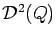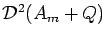Journal of Integer Sequences, Vol. 21 (2018), Article 18.4.4

## Relations between Powers of Dedekind Numbers and Exponential Sums Related to Them

### Frank a Campo Seilerwall 33 D-41747 Viersen Germany

Abstract:

Letdenote the downset-lattice of the downset-lattice of the finite poset Q and let d2(Q) denote the cardinality of. We investigate relations between the numbers d2(Am + Q) and their powers, where Am is the antichain with m elements and Am + Q the direct sum of Am and Q. In particular, we prove the inequality d2(Q)3 < d2(A1 + Q)2 based on the construction of a one-to-one mapping between sets of homomorphisms. Furthermore, we derive equations and inequalities between the numbers d2(Am + Q) and exponential sums of downset sizes and interval sizes related to. We apply these results in a comparison of the computational times of algorithms for the calculation of the Dedekind numbers d2(Am), including a new algorithm.

Full version:  pdf,    dvi,    ps,    latex,     Corrigendum

(Concerned with sequence A000372.)

Received January 20 2018; revised versions received May 1 2018; May 2 2018. Published in Journal of Integer Sequences, May 8 2018. Corrigendum, October 26 2020.